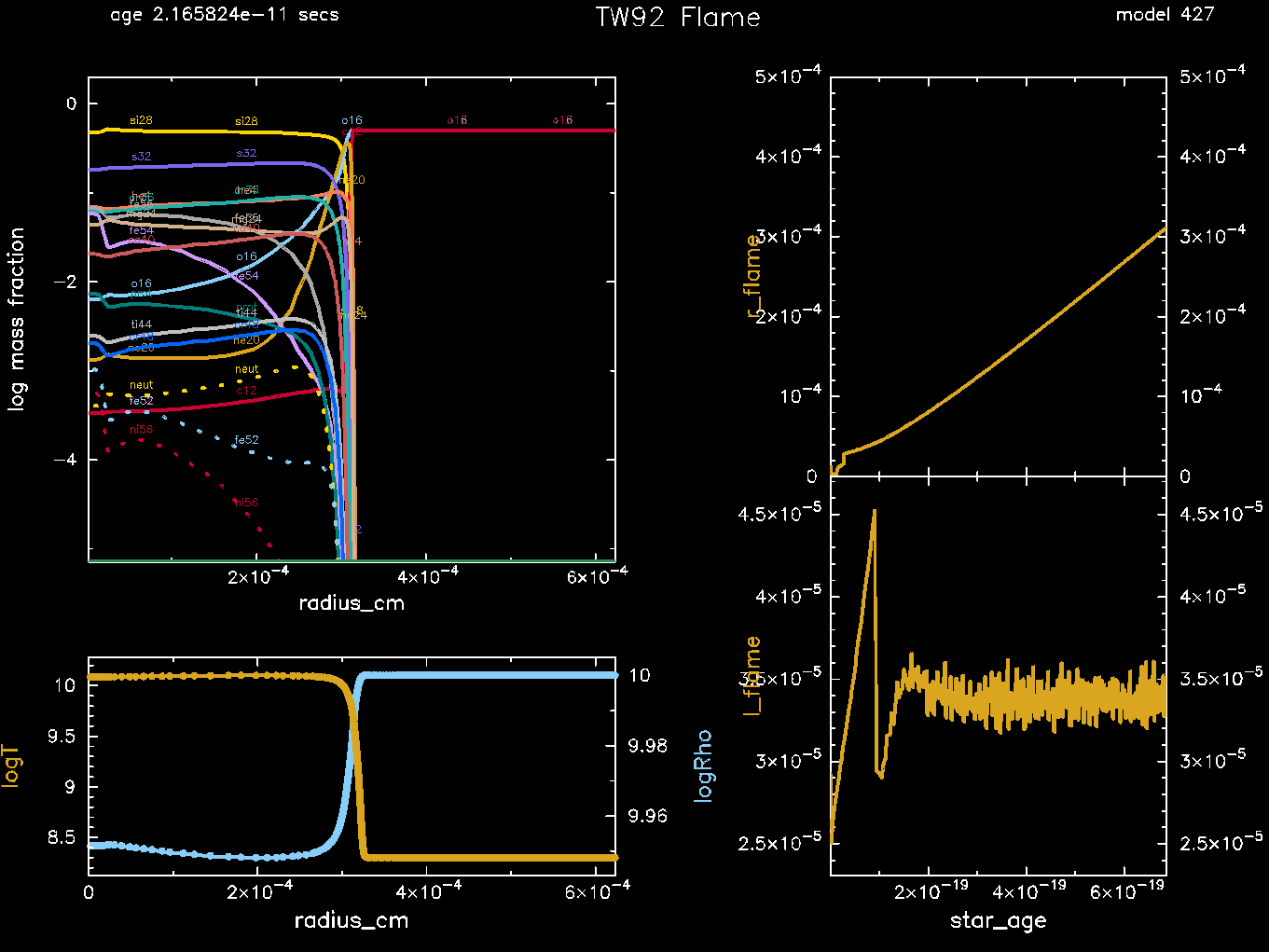# conductive_flame¶

This test suite case models a conductively-propagated deflagration wave (“flame”) in a high-density, degenerate carbon-oxygen mixture. It is similar to a calculation presented by Timmes & Woosley (1992).

Warning

Careful modeling of the flame speed requires a much larger nuclear network than the 21 isotope network used in this test. Timmes & Woosley (1992) use a 130 isotope network, Chamulak et al. (2007) use a 430 isotope network, and Schwab et al. (2020) use a 495 isotope network.

Unlike most MESA calculations, this models a small sphere of material (instead of an entire star). The initial model is built in run_star_extras using the other_build_initial_model hook. A spatially uniform model with a given density, temperature, and composition is constructed. A small hot spot is then added at the center of the model. The properties of this initial model can be controlled from the inlist.

      use_other_build_initial_model = .true.
x_integer_ctrl(1) = 300 ! number of points
x_ctrl(1) = 10 ! mass (in g)
x_ctrl(2) = 1d10 ! initial density (cgs)
x_ctrl(3) = 2d8 ! initial temperature (cgs)

x_ctrl(4) = 0.0001 ! size (in q) of region to heat
x_ctrl(5) = 5d9 ! temperature of that region

x_ctrl(6) = 0.50 ! mass fraction c12
x_ctrl(7) = 0.50 ! mass fraction o16


The inner boundary is at r = 0. The outer boundary has a fixed temperature and a fixed pressure equal to the initial pressure of the material. This is achieved via the use_other_surface_PT hook.

After an initial transient, the entire flame structure, approximately isobaric, propagates into the upstream fuel with a unique speed and width. The test succeeds if the flame successfully propagates through half of the domain and reports values for the flame width and speed that are within 10% of the specified reference values (see inlist). The flame width and speed are reported to the TestHub.

The flame speed is measured using the time/position when the flame is 30% of the way through the domain and time/position at the end of the test. The flame width is measured at the end of the test as the width over which eps_nuc is greater than 10% of its peak value. See routine flame_properties in the run_star_extras.f90.Last-Updated: 2021-06-21 (mesa b2364463) by Josiah Schwab